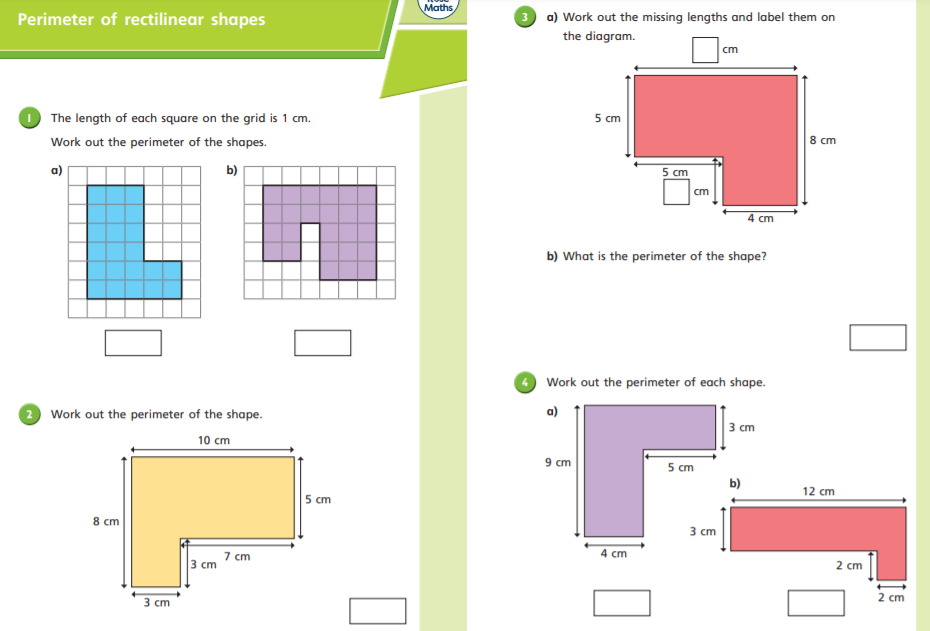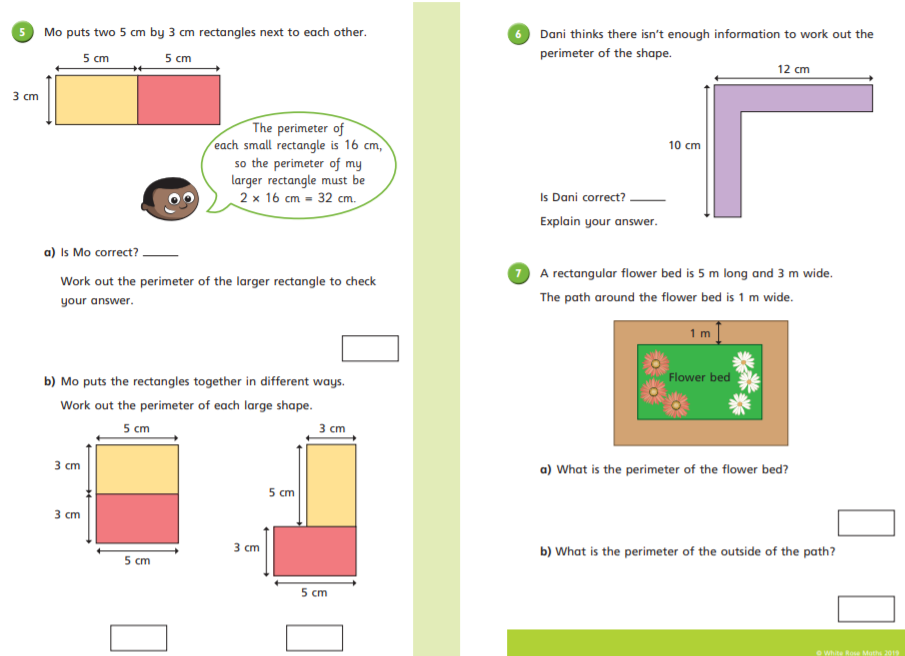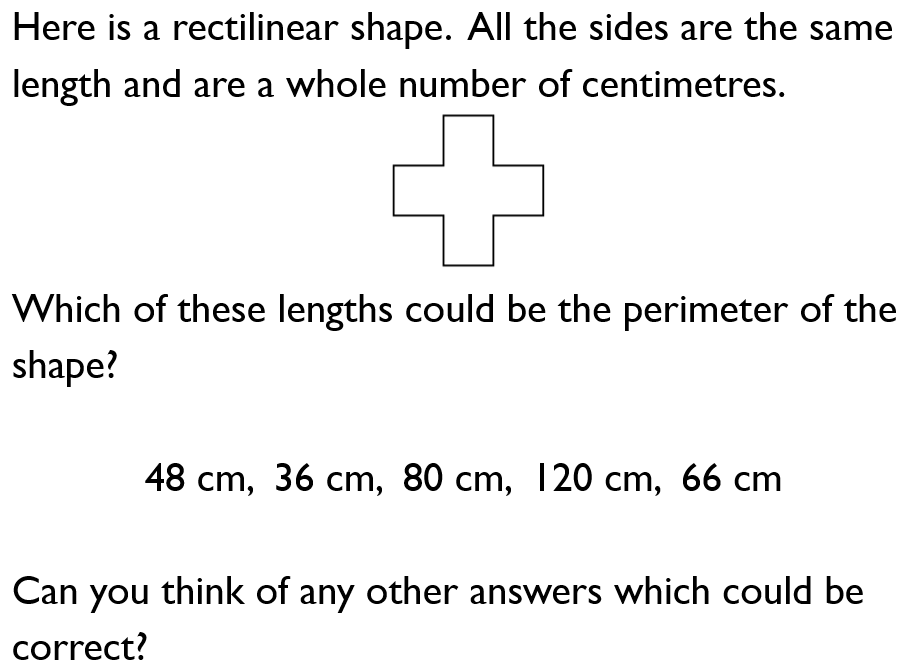# Maths – Thursday 25th February (Zoom at 2pm to mark the worksheet)

Today’s lesson is about finding the perimeter of rectilinear shapes.

A rectilinear shape is a shape that has straight sides and right angles. It can look like two rectangles have been joined together.

This shape looks much more complicated than a rectangle, but the method of working out the perimeter is the same. Add together the lengths of all sides to find it.

Pause and rewind the video whenever you need to.

Here is the worksheet to answer at different times during the video.CHALLENGE

Investigate this question about the perimeter of rectilinear shapes.### 20 thoughts on “Maths – Thursday 25th February (Zoom at 2pm to mark the worksheet)”

•25th February 2021 at 9:38 am

maths:
1. 22cm
b. 25cm
2. 36cm
3. a. 10cm 2cm I don’t really know
b. 34cm
4. a. 30cm
b. 34cm
5. a. yea he’s right
b. 22cm 24cm
6. no because just work out the last few
7. 16m
b. 1m?

•25th February 2021 at 2:47 pm

Good effort Kiran

The ones to check are:

1b, 3a (use the other sides to work out the missing ones), 4a, 5a, 5b (second shape), 7b.

•25th February 2021 at 9:55 am

1) a) 22cm b) 26cm
2) 36cm
3) a) 9cm and 3cm b) 34cm
4) a) 30cm b) 24cm
5) a) NO 26cm b) 22cm 24cm
6) Yes, without the bottom side labeled you wouldn’t be able to do the side under the top
7) a) 16m b) 20m

•25th February 2021 at 2:48 pm

Good effort Franek.

The ones to check are:

4a, 5b (second shape), 6, 7b.

•25th February 2021 at 10:09 am

36 cm?

•25th February 2021 at 2:49 pm

Which question is this for Rihanna?

•25th February 2021 at 11:00 am

1. 21, 26
2. 36
3. 9cm, 3cm-34cm
4. 36cm, 29cm
5. Yes, 16+16 as 8+8.
6. Yes, because the information won’t let you find the perimeter and the sides.
7. 16, 24

Challenge
36cm because the sizes are very small.

•25th February 2021 at 2:50 pm

Well done Hargun.

The ones to check are:

1a, 5a-c, 6.

•25th February 2021 at 11:03 am

worksheet: it wot let me print the sheet.

challenge: done

•25th February 2021 at 2:51 pm

Ok. You can still work from the screen. I will also add the worksheet images to see if that helps.

Mr Bushell

•25th February 2021 at 12:35 pm

1)
5+5+9+1+7+3+=30

2)
28mm
3)
26-13=13
4)
57=66-9

2+3+3+2+5+5=20cm
7+6+4+5+3+1=31cm
2+3+5+5+7+8=30cm

14+9+6+10+3+4=46cm

5+5+5+5+3+3=26

•25th February 2021 at 2:53 pm

For the questions on perimeter (not the Get Ready section):

Check that you have worked out the length of each side and added them together carefully.

Mr Bushell

•25th February 2021 at 2:41 pm

1a 22 cm b 26cm
2 36 cm
3 9 and 3 B 34 cm
4 a 36 cm b 34 cm
5 no b 26 cm
6 no

•25th February 2021 at 2:55 pm

You probably know these are right because we just did them together on the Zoom!

Next time, make sure you’ve had a go first. It’s not a problem if you get them wrong – we learn from our mistakes.

•25th February 2021 at 2:48 pm

Maths work sheet

1.a.=22cm
b.=26cm
3.=36cm
4.a.=36cm
b.34cm
5.a.=36cm
b.=21 and 24
6.=No
7. I don’t know a and b.

•25th February 2021 at 2:58 pm

Hello Mia.

The ones to check are:
Q3, 5 a and b.

For Q7, the measurements for the flower bed are in the writing and question. You will need to find it and then use it correctly. Give it a go!

Mr Bushell

•25th February 2021 at 3:14 pm

maths

1.
A) 22cm
B) 26 cm

2.
36cm

3.
3cm and 9cm

4.
a) 30 (added the 9 cm on)
b) 29 (added the 10 cm on)

5.
Mo is not correct

a)the perimeter is 26 cm.
B) they are both 26 cm

6.
Dani is correct

7.
a) 16 m
b) 24 m

•25th February 2021 at 3:44 pm

Well done Owen.

Check question 4 (a and b), 5a and 6.

Mr Bushell

•25th February 2021 at 5:22 pm
•